# Fractions

Sort fractions
${z}_{1}=\frac{6}{11};{z}_{2}=\frac{10}{21};{z}_{3}=\frac{19}{22}$
by its size. Result write as three serial numbers 1,2,3.

n(z1):  2
n(z2):  1
n(z3):  3

### Step-by-step explanation: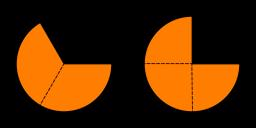Did you find an error or inaccuracy? Feel free to write us. Thank you!Tips to related online calculators
Do you want to calculate least common multiple two or more numbers?
Need help to calculate sum, simplify or multiply fractions? Try our fraction calculator.

## Related math problems and questions:

• LCD 2The least common denominator of 2/5, 1/2, and 3/4
• Paper collecting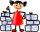At the paper collecting contest gathered Franta 2/9 ton, Karel 1/4 ton, and Patrick 19/36 tons of paper. Who has gathered the most and the least?
• CherriesCherries in the bowl can be divided equally among 19 or 13 or 28 children. How many are the minimum cherries in the bowl?
• Expression plus minusEvaluate expression: (-1)2 . 12 – 6 : 3 + (-3) . (-2) + 22 – (-3) . 2
• Buses 4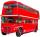intervals: 1st bus 40 min. 2nd bus 2h 3rd bud 20min How long take them to meet - as soon as possible?
• LCMCommon multiple of three numbers is 3276. One number is in this number 63 times, second 7 times, third 9 times. What are the numbers?
• Lcm 2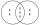Create the smallest possible number that is divisible by numbers 5,8,9,4,3
• Lcm of three numbersWhat is the Lcm of 120 15 and 5
• Ten fractionsWrite ten fractions between 1/3 and 2/3
• Fractions and mixed numerals(a) Convert the following mixed numbers to improper fractions. i. 3 5/8 ii. 7 7/6 (b) Convert the following improper fraction to mixed number. i. 13/4 ii. 78/5 (c) Simplify these fractions to their lowest terms. i. 36/42 ii. 27/45 2. evaluate following ex
• Lcm simpleFind least common multiple of this two numbers: 140 175.Why does 1 3/4 + 2 9/10 equal 4.65? How do you solve this?The table is given: days complaints 0-4 2 5-9 4 10-14 8 15-19 6 20-24 4 25-29 3 30-34 3 1.1 What percentage of complaints were resolved within 2weeks? 1.2 calculate the mean number of days to resolve these complaints. 1.3 calculate the modal number of dayWhat is the least common denominator of 11/15 and 12/19?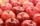How many minimum apples are in the cart, if possible is completely divided into packages of 6, 14 and 21 apples?2/3x + 5/7 = 1/2x + 22/21Add two mixed fractions: 2 4/6 + 1 3/6